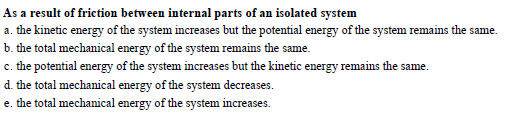# As a result of friction between internal parts of an isolated systema, the kinehic energy of the system increases h nergy of the system remins the sameb. the total mechanical energy of the system remains the same.c. the potential energy of the system increases but the kinetic energy remains the same.system decreases.e. the total mechanical energy of the system imcreases.

Question
388 viewshelp_outlineImage TranscriptioncloseAs a result of friction between internal parts of an isolated system a, the kinehic energy of the system increases h nergy of the system remins the same b. the total mechanical energy of the system remains the same. c. the potential energy of the system increases but the kinetic energy remains the same. system decreases. e. the total mechanical energy of the system imcreases. fullscreen
check_circle

Step 1

Mechanical energy is the sum of kinetic and potential energy of an object.

Step 2

When two surfaces are rubbed, due to friction, the kinetic energy will get converted to thermal energy. Thus a result the kinetic energy will decrease.

Step 3

In case of friction between internal parts of an isolated system, the kinetic energy will get converted to thermal energy, reducing the kinetic energ...

### Want to see the full answer?

See Solution

#### Want to see this answer and more?

Solutions are written by subject experts who are available 24/7. Questions are typically answered within 1 hour.*

See Solution
*Response times may vary by subject and question.
Tagged in

### Work,Power and Energy